音影片學習之路--C++

前言

C和C++作為學習音影片技術首要具備的語言基礎，所以十分必要學習和複習一下之前學習C++語言基礎。

正文

C++作為一門用途更廣、功能更齊全的語言，其知識深度很深，所以這裡也就複習、學習一些基本知識點，等後續在實際專案中有遇到難點再進行補充。

include

//名稱空間，告訴編譯器使用std名稱空間 using namespace std;

int main() { printf("Hello, World! \n"); //這裡的cout就是std裡面定義的函式 //<<是操作符過載，後面細說 cout << "Hello, World!" << endl; return 0; } ``` 列印是：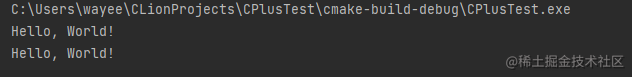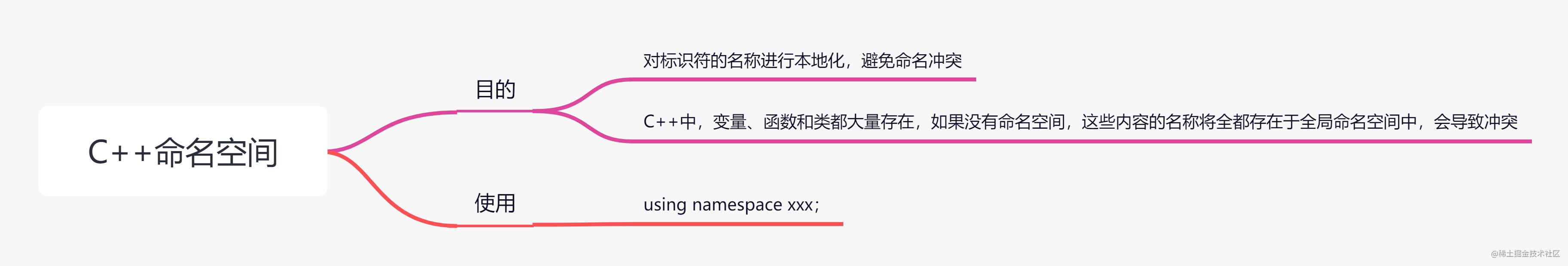關鍵字

C++關鍵字有很多，我們不能和學習C語言一樣，全都羅列一遍給看一下，這裡就看一些常用的，後面有用到其他的再進行補充。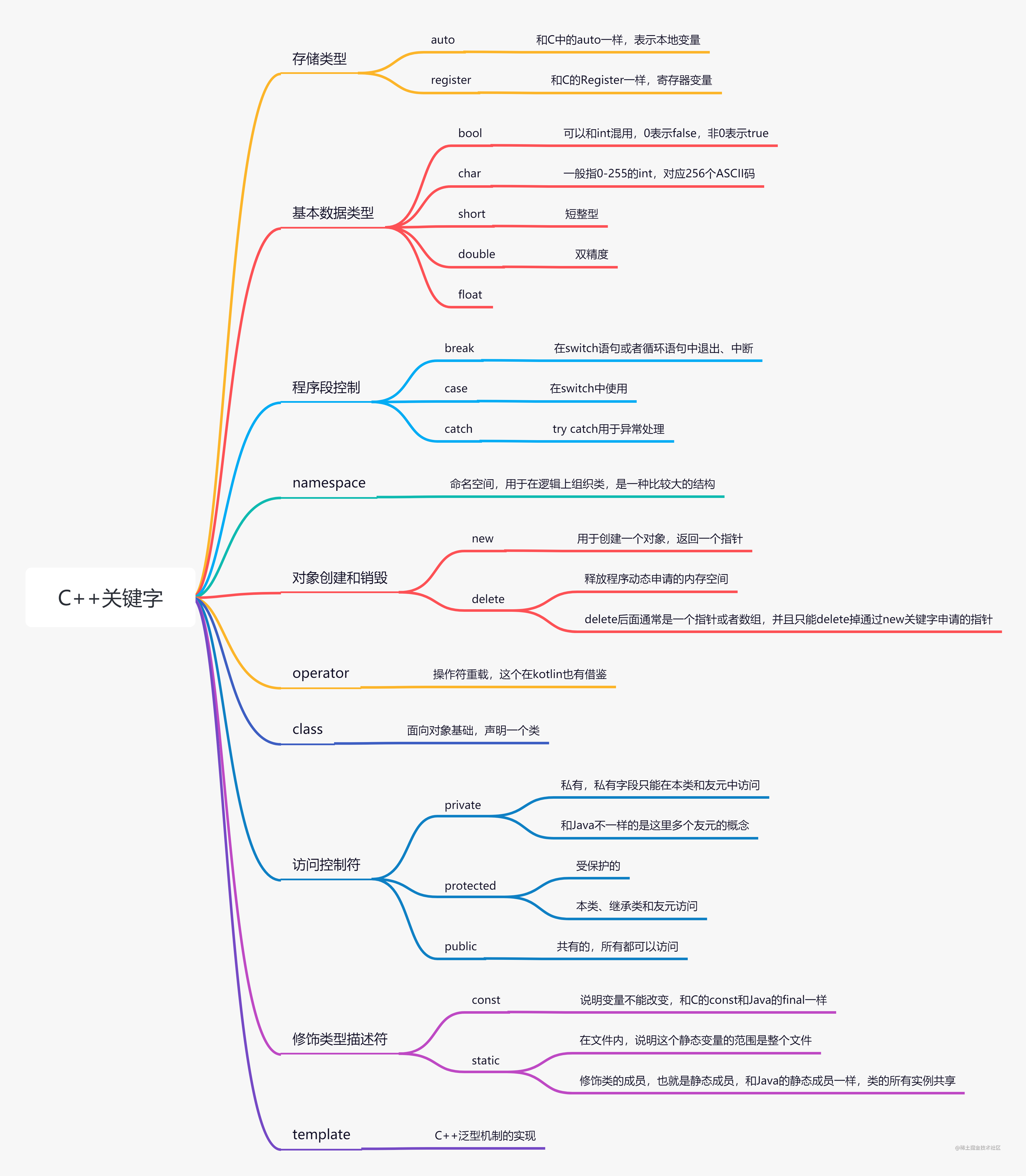變數作用域

• 在函式或者一個程式碼塊內部宣告的變數，叫做區域性變數。

• 在函式引數的定義中宣告的變數，叫做形式引數。

• 在所有函式外部宣告的變數，叫做全域性變數。

定義常量

• 使用#define前處理器。

• 使用const關鍵字。

//這個必須得有

include

using namespace std;

define NAME "zyh"

const int AGE = 20;

const string COMPANY = "WY";

int main() { cout << NAME << endl; cout << "age : " << AGE << endl; cout << "company : " << COMPANY << endl; return 0; } ``` 列印結果：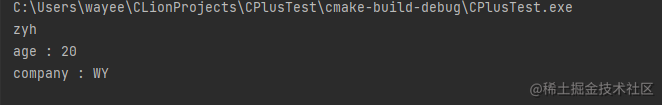字串

``` using namespace std;

int main() { //C風d格定義字串 char greet = "Hello"; char *greet1 = "Hello"; char greet2[] = "Hello"; //C++使用string型別 string greet3 = "Hello";

cout << greet << endl;
cout << greet1 << endl;
cout << greet2 << endl;
cout << greet3 << endl;

return 0;

} ```

指標

• 簡化一些任務，使用指標
• 動態記憶體分配，當動態記憶體分配時，必須要使用指標

引用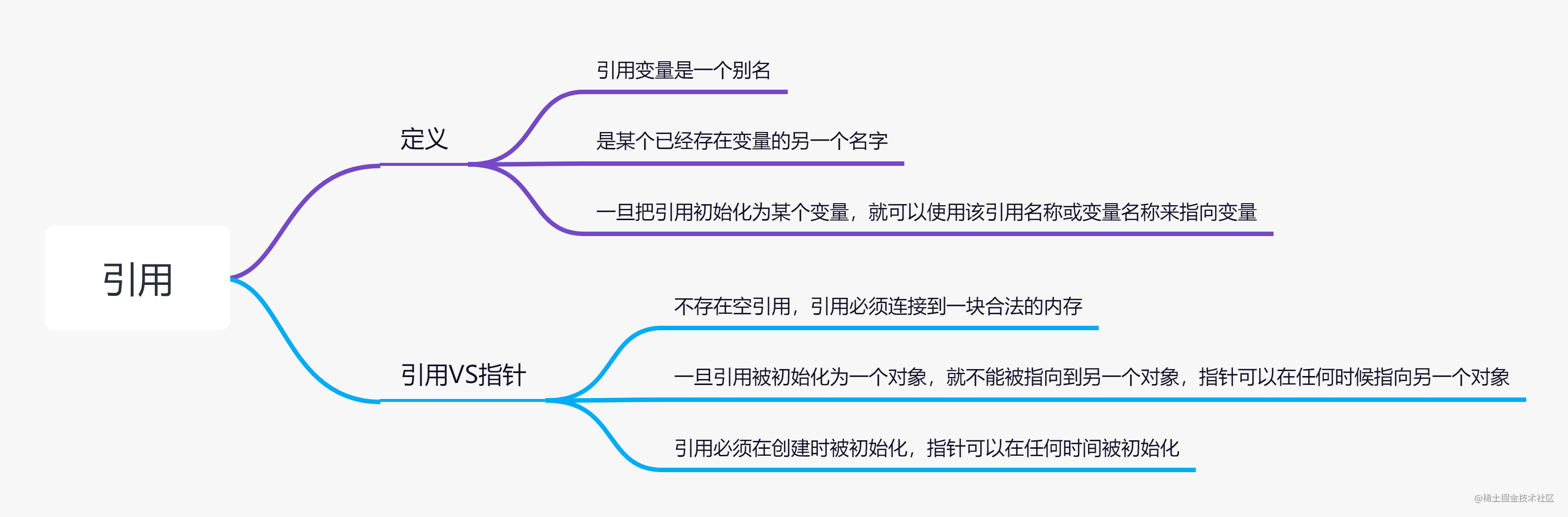``` using namespace std;

int main() { //宣告變數 int a = 10,b = 20; //宣告引用變數 int &i = a; int &j = b;

cout << "a ==" << a << "\t &i ==" << i <<endl;
cout << "b ==" << b << "\t &j ==" << j <<endl;
//改變變數的值，引用也會變化
a = 5,b = 6;

cout << "a ==" << a << "\t &i ==" << i <<endl;
cout << "b ==" << b << "\t &j ==" << j <<endl;

return 0;

} ``` 這裡聲明瞭引用，也就相當於a和i指向同一塊記憶體區域，但是它可以和a一樣使用，也就相當於變數，其地址是一樣的，列印如下：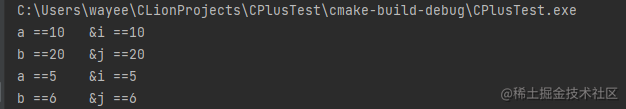函式引數為引用型別

``` using namespace std;

void swap(int& x,int& y);

void swap1(int x,int y);

int main() { int a = 100,b = 200; cout << "交換前 a = " << a << " b = " << b << endl; swap(a,b); //發生了變化，函式執行影響了傳遞的引數值 cout << "第一次交換後 a = " << a << " b = " << b << endl; swap1(a,b); //沒有發生變化，值傳遞會複製引數，不會影響 cout << "第二次交換後 a = " << a << " b = " << b << endl;

return 0;

} //引數是引用型別 void swap(int& x,int& y){ int temp; temp = x; x = y; y = temp; } //引數是值 void swap1(int x,int y){ int temp; temp = x; x = y; y = temp; } ```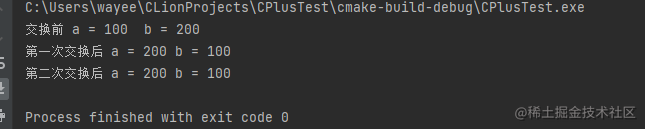```

define CPLUSTEST_PERSON_H

class Person{ //公共屬性 public: Person(); //空引數建構函式 ~Person(); //解構函式 Person(char *name,int age); //有參建構函式

//成員變數
char *name;
int age;

//函式
void setName(char *name);
char *getName();

void setAge(int age);
int getAge();

};

endif //CPLUSTEST_PERSON_H

``` 這是person.h檔案，前面在學習C語言種說過，標頭檔案一般是定義函式、類啥的，這裡也一樣，不過這裡僅僅只是定義，和Java還是有著非常大的區別，真正實現的地方在下面：

```

include "person.h"

using namespace std;

Person::Person(char *name, int age) { this -> name = name; this -> age = age; }

Person::~Person() { cout << "Person銷燬" << endl; }

Person::Person() { cout << "執行 Person 空建構函式" << endl; }

void Person::setAge(int age) { this -> age = age; }

void Person::setName(char *name) { this -> name = name; }

int Person::getAge() { return this -> age; }

char *Person::getName() { return this -> name; } 這個是person.cpp檔案，匯入前面標頭檔案，這裡是進行實現，這裡和Java的類定義區別很大，在Java或者kotlin中一般定義後就直接都實現了，不會單獨搞2個地方，然後就是呼叫的地方： int main() {

//棧裡面定義的 在該方法執行完就會回收掉Person物件
Person personTemp;
personTemp.setName("張三");
personTemp.setAge(10);
cout << personTemp.getName() << "\t" << personTemp.getAge() << endl;

//如果使用new初始化建構函式，
Person *person = new Person("zyh",18);
cout << person -> name << "\t" << person->getAge() << endl;
//釋放person記憶體
delete person;

return 0;

} ``` 同樣這裡也有著很大的區別，首先是物件宣告，在Java中一個物件如果沒有呼叫建構函式它就是null，無法呼叫其方法，但是在C++中確不一樣，同時使用new操作符新建的物件，需要手動delete釋放記憶體，Java是有GC自動回收，也比較麻煩。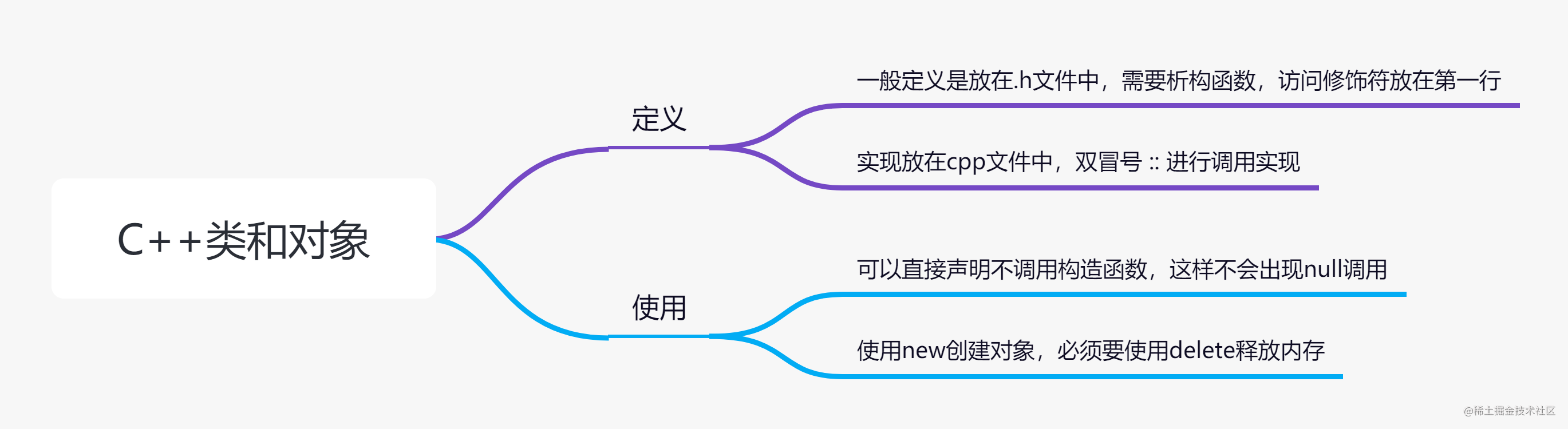define CPLUSTEST_BOX_H

//第一種是類成員函式直接在類中就定義

class Box{ public: double length; //長度 double width; //寬度 double height; //高度

double getVolume(){
return length * width * height;
}

double getVolume2();    //體積

};

include "box.h"

double Box::getVolume2() { return length * width * height * 2; } ``` 上面2種方式都可以。其中重點說明一下在類中定義的成員函式把函式宣告為內聯的，啥是內聯，後面再說；其中 :: 叫做範圍解析運算子。

```

define CPLUSTEST_BOX_H

//第一種是類成員函式直接在類中就定義

class Box{ public: double length; //長度 double width; //寬度 double height; //高度

double getVolume(){
return length * width * height;
}

double getVolume2();    //體積
//自定義的建構函式
Box(double len);

};

endif //CPLUSTEST_BOX_H

``` int main() {

//棧裡面定義的 在該方法執行完就會回收掉Person物件
Person personTemp;
personTemp.setName("張三");
personTemp.setAge(10);
cout << personTemp.getName() << "\t" << personTemp.getAge() << endl;

//如果使用new初始化建構函式，
Person *person = new Person("zyh",18);
cout << person -> name << "\t" << person->getAge() << endl;
//釋放person記憶體
delete person;

return 0;

} ``` 列印如下: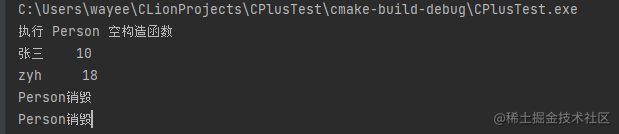```

define CPLUSTEST_LINE_H

class Line{ public: int getLength(); Line(int len); //拷貝建構函式，必須要定義 Line(const Line &obj); ~Line();

private: //這個是指標變數 int *ptr; };

include

using namespace std;

Line::Line(int len) { cout << "呼叫建構函式" << endl; //由於傳遞進來的是個int值，所以要先給指標分配記憶體 ptr = new int ; //指標指向的記憶體值是len *ptr = len; }

Line::Line(const Line &obj) { cout << "呼叫拷貝建構函式" << endl; //這裡拷貝的時候就需要深拷貝了，新的物件的指標要重新分配記憶體 ptr = new int ; //指標指向的值進行賦值 ptr = obj.ptr; }

Line::~Line() { cout << "釋放記憶體" << endl; delete prt; } ``` 從上面程式碼我可以看出一個問題，就是C++的淺拷貝和深拷貝問題，和Java一樣，如果是這種指標變數，需要重新分配記憶體。

define CPLUSTEST_BOX_H

class Box{ double width; //預設是私有變數 public: friend void printWidth(Box box); //宣告為友元函式 void setWidth(double width);

};

endif //CPLUSTEST_BOX_H

``` 這裡聲明瞭一個友元函式，用來獲取私有變數的，

```

include "box.h"

using namespace std;

void Box::setWidth(double width) { this->width = width; }

void printWidth(Box box){ cout << "width = " << box.width << endl; } 友元函式因為不是Box中的，所以不能使用Box::來呼叫，完成定義後，便可以使用了： using namespace std;

int main() {

Box *box = new Box();
box->setWidth(10);
printWidth(*box);
delete box;

} ``` 感覺有點神奇，這個width屬性沒有任何get方法對外，居然可以通過友元訪問到，不得不說C++的設計很到位。

「其他文章」